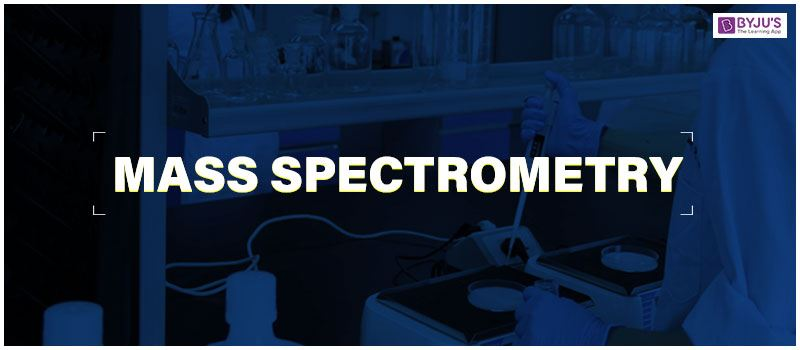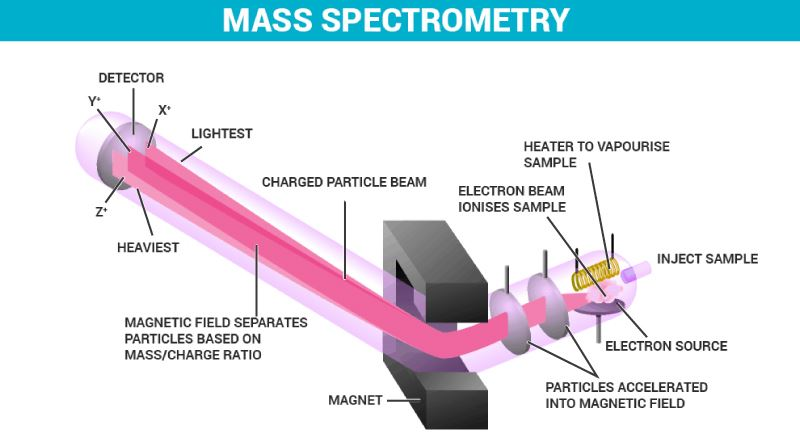# Mass SpectrometryMass spectrometry

Ever wondered how forensic scientists analyze the smallest of ounces of evidence they find in a crime scene? Well, with the help of mass spectrometry you can even find out about the different isotopes associated with that element. Let us learn a bit more about Mass Spectrometry.

## What is Mass Spectrometry?

Mass spectrometry is an analytical method to find the molecular mass of a compound and indirectly helped to prove the identity of isotopes.

### 1. Principle of Mass Spectrometry

Based on Newton’s second law of motion and momentum, a mass spectrometer uses this property of matter to plot ions of varying masses on a mass spectrum. From the law, we infer how much mass is relevant to the inertia and acceleration of a body. This principle is applied to the aspect where ions with different mass to charge ratios are deflected by different angles in an electric or magnetic field.

### 2. Mass Spectrum

A mass spectrum is a graph obtained by performing mass spectrometry. It is a relation between the mass to charge ratio and ion signal.

### 3. Mass Spectrometry Diagram

• Inlet system
• Ionization
• Deflector
• Ion detectorMass Spectrometry Instrumentation

## Mass Spectrometry Instrumentation:

The four main parts of mass spectrometry are discussed below:

1. Ionizer – The bombarding of the sample is done by the electrons. These electrons move between cathode and anode.  When the sample passes through the electron stream between the cathode and anode, electrons with high energy knock electrons out of the sample and form ions.
2. Accelerator – The ions placed between a set of charged parallel plates get attracted to one plate and repel from the other plate. The acceleration speed can be controlled by adjusting the charge on the plates.
3. Deflector – Magnetic field deflects ions based on its charge and mass. If an ion is heavy or has two or more positive charges, then it is least deflected. If an ion is light or has one positive charge, then it is deflected the most.
4. Detector – The ions with correct charge and mass move to the detector. the ratio of mass to charge is analyzed through the ion that hits the detector.

## How does Mass Spectrometry Work?

In a regular mass spectrometer, we initially have the material to be analyzed, but we need it to be ionized to pass through the spectrometer with enough energy. Thus, the sample is bombarded by electrons to ionize it.

This ionized beam is now passed through a series of electric or magnetic fields depending on the type of the sample and its properties. The ions are deflected by the field through which they are passed through in such a way that the ions with the same mass to signal ratio will follow the same path to the detector.

These charged and deflected ions are now incident onto a detector which is capable of distinguishing the charged particles falling on it. Based on the mass spectrum produced by the charged ions, we can identify the atoms or molecules constituting the sample by comparing them with known masses or through a characteristic fragmentation pattern.

## Applications of Mass Spectrometry

• Due to its capability to distinguish between substances, Mass spectrometry is used to determine unknown substances.
• To identify the isotopes of a substance.
• In analytical laboratories that study the chemical, physical and biological properties of substances. It is favored over several other analytical techniques as it has less background interference since it is performed in a vacuum.

One disadvantage of mass spectrometry is over hydrocarbons. The method fails to distinguish between hydrocarbons producing similarly fragmented ions.

Thus, here we have seen a brief description of what a mass spectrometer is, its structure and how it functions.

## FAQs

1. What is the basic principle of mass spectrometry?
Ans: A mass spectrometer generates multiple ions from the sample being investigated, then separates them by their specific mass-to-charge ratio (m/z) and then records the relative abundance of each type of ion.

2. What are the applications of mass spectrometry?
Ans: Mass spectrometry is a powerful technique with many different applications in biology, chemistry, and physics, but also in clinical medicine and even in space exploration. It is used by separating molecular ions on the basis of their mass and charge to determine the molecular weight of compounds.

3. What is mass accuracy in mass spectrometry?
Ans: Mass precision is a metric that defines the difference between an ion’s measured mass/charge (m/Q) and that ion’s true, exact m/Q. The DAQ records mass accuracy in parts per million (ppm) for each of the three input calibration ions.

4. Is mass spectrometry quantitative or qualitative?
Ans: Design and application of mass spectrometry in biological fluids, tissues, species or cells for the qualitative and quantitative study of low molecular compounds and macromolecules.

5. What is unit mass resolution?
Ans: Unit resolution means that the next integer mass can be separated from each volume. That is, mass 50 can be distinguished from mass 51, and mass 1000 from mass 1001 can be distinguished. This concept is widely used to describe resolution on mass spectrometers of quadrupole and ion trap.